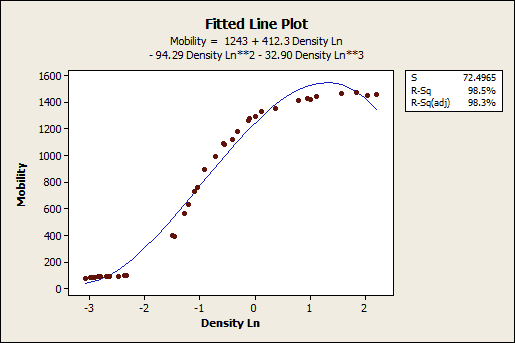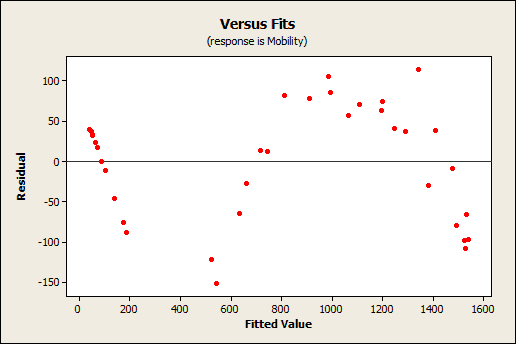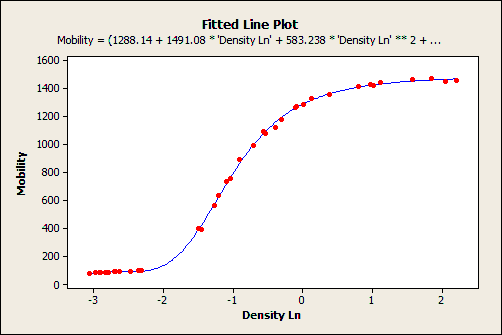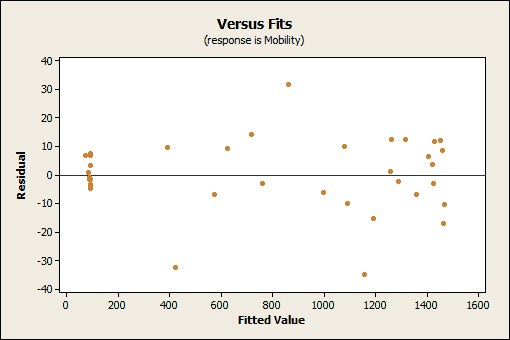As you probably noticed, the field of statistics is a strange beast. Need more evidence? Linear regression can produce curved lines and nonlinear regression is not named for its curved lines.

So, when should you use Nonlinear Regression over one of our linear methods, such as Regression, Best Subsets, or Stepwise Regression?

Generally speaking, you should try linear regression first. It’s easier to use and easier to interpret.  However, if you simply aren’t able to get a good fit with linear regression, then it might be time to try nonlinear regression.

Let’s look at a case where linear regression doesn’t work. Often the problem is that, while linear regression can model curves, it might not be able to model the specific curve that exists in your data.  The graphs below illustrate this with a linear model that contains a cubed predictor.The fitted line plot shows that the raw data follow a nice tight function and the R-squared is 98.5%, which looks pretty good. However, look closer and the regression line systematically over and under-predicts the data at different points in the curve. When you check the residuals plots (which you always do, right?), you see patterns in the Residuals versus Fits plot, rather than the randomness that you want to see. This indicates a bad fit, but it’s the best that linear regression can do.

Let’s try it again, but using nonlinear regression. It's important to note that because nonlinear regression allows a nearly infinite number of possible functions, it can be more difficult to setup. In this case, it required considerable effort to determine the function that provided the optimal fit for the specific curve present in these data, but since my main point is to explain when you want to use nonlinear regression instead of linear, we don't need to relate all of those details here. (Just like on a cooking show, on the blog we have the ability to jump from the raw ingredients to a great outcome in the graphs below without showing all of the work in between!)

What is the difference between linear and nonlinear regression equations?The fitted line plot shows that the regression line follows the data almost exactly -- there are no systematic deviations. It’s impossible to calculate R-squared for nonlinear regression, but the S value (roughly speaking, the average absolute distance from the data points to the regression line) improves from 72.4 (linear) to just 13.7 for nonlinear regression. You want a lower S value because it means the data points are closer to the fit line. What's more, the Residual versus Fits plot shows the randomness that you want to see.  It’s a good fit!

Nonlinear regression can be a powerful alternative to linear regression but there are a few drawbacks. In addition to the aforementioned difficulty in setting up the analysis and the lack of R-squared, be aware that:

• The effect each predictor has on the response can be less intuitive to understand.
• P-values are impossible to calculate for the predictors.
• Confidence intervals may or may not be calculable.

If you're using Minitab now, you can play with this data yourself by going to File -> Open Worksheet, then click on the Look in Minitab Sample Data folder icon and choose Mobility.MTW. These data are the same that I’ve used in the Nonlinear Regression Help example, which contains a fuller interpretation of the Nonlinear Regression output.

If you'd like to try it, you can download the free 30-day trial of Minitab Statistical Software. If you're learning about regression, read my regression tutorial!